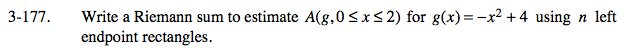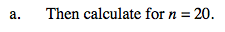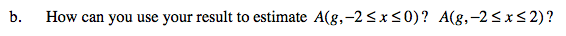### Home > CALC > Chapter 3 > Lesson 3.4.3 > Problem3-177

3-177.
1. Write a Riemann sum to estimate A(g, 0 ≤ x ≤ 2) for g(x) = −x2 + 4 using n left endpoint rectangles. Homework Help ✎

1. Then calculate for n = 20.

2. How can you use your result to estimate A(g, −2 ≤ x ≤ 0)? A(g, −2 ≤ x ≤ 2)?General left-endpoint Riemann sum:Substitute.Consider the shape of the graph of g(x).